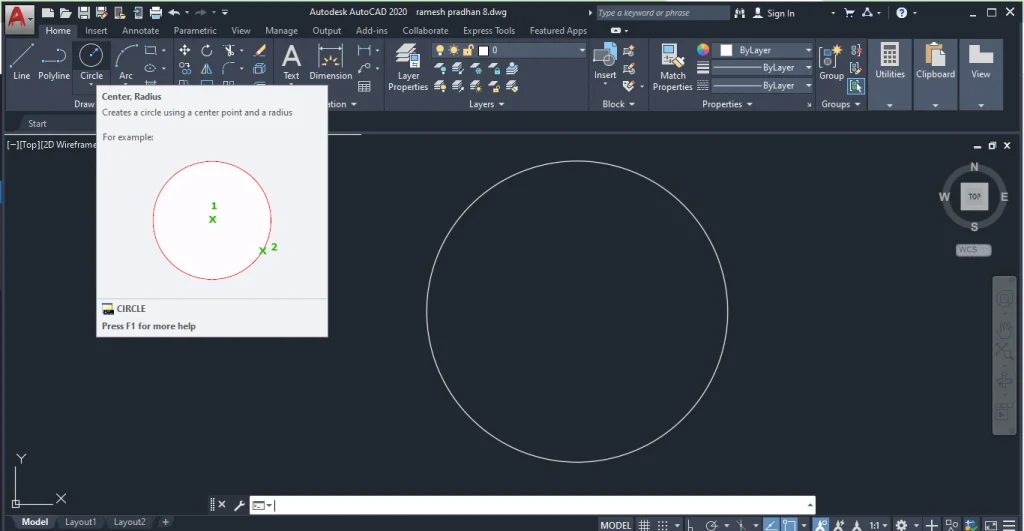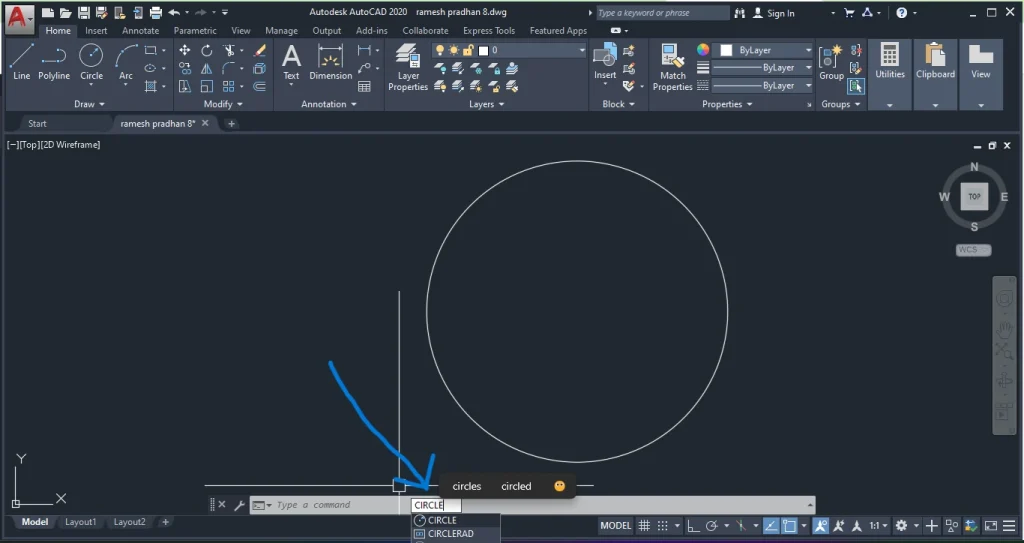# How to Divide a Circle in AutoCAD? Top Three Best Method

## Divide a Circle in AutoCAD

Hello guys welcome to the blog. If you are AutoCAD Drafter or Designer you have these basic tips to divide a circle in AutoCAD. If you want to divide a circle into equal parts follow the following ways. This article discusses the different methods to divide the circle in AutoCAD. After you get the Three best methods to divide the circle into equal parts to create a more integrated Design in AutoCAD. So let’s get started:

I am already discussed in detail of AutoCAD. Now I am introducing short information about AutoCAD. AutoCAD is a computer-aided design software that allows you to create and modify 2D and 3D designs, Which is developed by Autodesk inc company. The software is mostly used by the architect, engineers, Drafters, and marketers. This is very useful and the latest software in the world due to its accuracy and result oriented.

I hope you already know of the circle command. Before you divide a circle into equal parts in Autocad you need to know of circle command in AutoCAD. If you are an engineer or architect AutoCAD is very essential. when you use Autocad you can draw many times of circle in Autocad for different object creation.

The circle is created by using the shortcut command ‘C’. The circle command is used to create a circle by the specified radius and center point of the circle. You can also use ribbon and command prompt to create a circle object in AutoCAD. The creation of a circle is not a hard task, you can easily draw a circle. Now we go to the process of creating a circle by using the command prompt and ribbon:

### Using the Ribbon1. Open AutoCAD and select the “Home” tab from the ribbon.
2. Click on the “Circle” command in the “Draw” panel.
3. Specify the center point of the circle by clicking on the screen or typing in the coordinates.
4. Specify the radius of the circle by clicking on the screen or typing in the value.
5. Press “Enter” to create the circle.

### Using Command prompt1. Open AutoCAD and type in “Circle” in the command prompt.
2. Press “Enter.”
3. Specify the center point of the circle by clicking on the screen or typing in the coordinates.
4. Specify the radius of the circle by clicking on the screen or typing in the value.
5. Press “Enter” to create the circle.

After you create a circle you may go to the next steps to divide the circle into equal parts in AutoCAD;

## How to Divide the circle into equal parts?

I am including the best three methods to divide a circle in AutoCAD. I hope you will understand easily. If you have any confusion while dividing please comment in the comment section below.  The Three methods are as follows:

### Using the DIVIDE Command

The DIVIDE command in AutoCAD allows you to divide an object into a specified number of equal parts. To divide a circle using the DIVIDE command, follow these steps:

1. Create a circle using the circle command.
2. Type in “DIVIDE” in the command prompt.
3. Press “Enter.”
4. Select the circle you want to divide.
5. Enter the number of segments you want to divide the circle into.
6. Press “Enter.”

### Using the MEASURE Command

The MEASURE command in AutoCAD allows you to measure the distance between two points or the angle between two lines. This Command works in the distance and angle of the divided object. To divide a circle in AutoCAD using the MEASURE command, follow these steps:

1. Create a circle using the circle command.
2. Type in “MEASURE” in the command prompt.
3. Press “Enter.”
4. Select the center point of the circle.
5. Select a point on the circumference of the circle.
6. Type in the angle between two points (360/n, where n is the number of segments you want).
7. Press “Enter.”

### Using the ARRAY Command

I already discuss the ARRAY command in AutoCAD. This command helps to create a pattern of objects in a specified number of rows and columns in AutoCAD. So If you want to divide a circle using this command follow the following steps:

1. Create a circle using the circle command.
2. Type in “ARRAY” in the command prompt.
3. Press “Enter.”
4. Select the circle you want to divide.
5. Type in “P” for “Polar Array.”
6. Enter the number of segments you want to divide the circle into.
7. Press “Enter.”

## Top 10 Best Tips for Dividing a Circle in AutoCAD

1. Select the circle you want to divide.
2. Type “DIVIDE” in the command line or find it in the “Draw” menu.
3. Specify the number of segments you want to divide the circle into.
4. Choose the point on the circle where you want to start the division.
5. Press enter to accept the default value of 0 for the angle.
6. Alternatively, specify the angle between each segment if you want to divide the circle unevenly.
7. Choose whether to create points, blocks, or both at each division point.
8. Specify the name and insertion point for the block if you choose to create one.
9. Press enter to complete the command and divide the circle.
10. Use the “PEDIT” command to edit the properties of the points or blocks if necessary.
11. DIVIDE, MEASURE, and ARRAY commands have different properties in the different design Requirements.
12. Check your Units, Precision, and angle before starting the divide a circle in AutoCAD.
13. Double-check after you divide a circle to require shape and accurate alignment.

## Conclusion

Dividing a circle in AutoCAD is the best skill to integrate Design Objects. You Can Divide a circle in AutoCAD via different methods using the DIVIDE, MEASURE, and ARRAY commands.  So You can use the above method to Divide a circle in AutoCAD into equal parts. I hope this article is very useful to you. If you have some queries about AutoCAD fill them free in the comment section. Also, you see some important tips in the article.

## FAQs

### Is it possible to divide a circle into an odd number of segments in AutoCAD?

Yes, the DIVIDE and ARRAY commands allow you to divide a circle into any number of segments, including odd numbers.

### Can I use the MEASURE command to divide other shapes besides circles?

Yes, the MEASURE command can measure angles between lines and create shapes based on those measurements.

### Can I undo the DIVIDE command in AutoCAD?

Yes, you can use the UNDO command or type “U” in the command prompt to undo the DIVIDE command.

### Can I divide a circle by its diameter instead of its radius?

Yes, you can use the MEASURE command and input the angle as 180/n, where n is the number of segments you want to divide the circle into.

### Can I use the DIVIDE and ARRAY commands with other shapes besides circles?

Yes, the DIVIDE and ARRAY commands can be used with other closed shapes, such as polygons and ellipses

This site uses Akismet to reduce spam. Learn how your comment data is processed.

error: Content is protected !!